# Measurement of Angles Examples

An angle can be defined as the rotation from the initial point to an endpoint of a ray. Angle measurement is the amount of rotation from the initial to an endpoint of a ray. The angle is said to be a positive angle if the rotation is clockwise and negative if the rotation is anticlockwise. Angles can be measured by various units.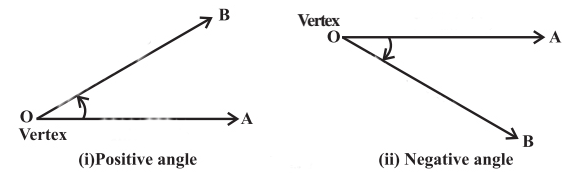## How to Measure an Angle?

The different systems for angle measurement are as follows:

• Measurement Of Angle – Degree Measure

A degree is defined as a complete rotation in either clockwise or anticlockwise direction, where the beginning and the ending point is the same. The rotation is divided into 360 units.

It is said to be 10 if the rotation from the initial and ending side is [1 / 360]th of the rotation. The degree is divided into hours, minutes and seconds. One degree is 60 minutes and one minute is 60 seconds.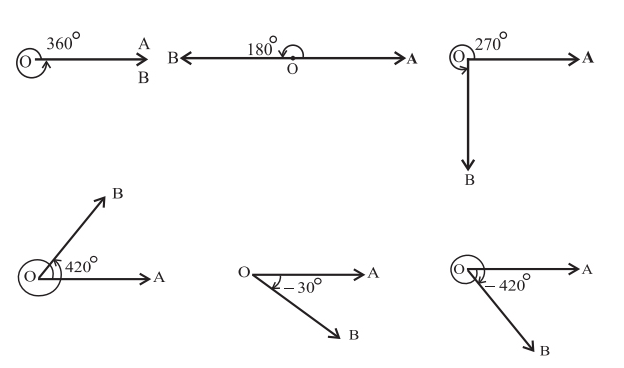• Measurement Of Angle – Radian Measure

Consider a circle of radius one unit. Let the arc of the circle be one unit. The measure of the angle is 1 radian if the arc subtends at the centre, provided the radius and arc lengths are equal.

The arc length of a circle with radius unity is equal to the angle in radian.

• Measurement Of Angle – Grade Measure

A grade can be defined as a right angle divided into a hundred equal parts. Further, each grade is divided into a hundred minutes and each minute into a hundred seconds.

## Measurement Of Angles Formula

The following formulae can be used in the measurement of angles.

Degree Measure

$1^0=[\frac{1}{360}]^{th}of \ a \ complete \ rotation$

$\theta =\frac{l}{r}$ where l is the arc length and r is the radius of the circle

It is known that a complete rotation in degrees is 3600 and 1 complete rotation = 2π radians in radian measure.

Assuming, π = 3.14159

$1 \ radian= \frac{180^0}{\pi} \\ 1 \ degree = \frac{\pi}{180}$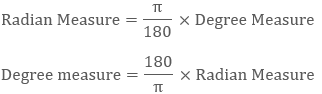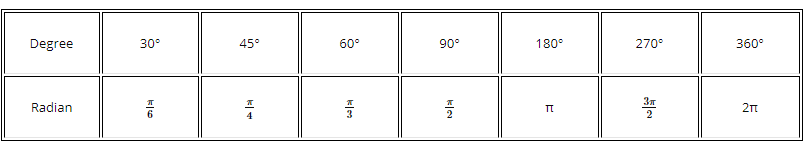Let D be the number of degrees, R be the number of radians and G be the number of grades in an angle $\theta , \text then \ \frac{D}{90}=\frac{G}{100}=\frac{2R}{\pi }$

This is the required relationship between the three systems of angle measurement.

Therefore, one radian $\cos A.\cos 2A.\cos {{2}^{2}}A.\cos {{2}^{3}}A…….\cos {{2}^{n-1}}A=\frac{\sin {{2}^{n}}A}{{{2}^{n}}\sin A},\,\text{if }A=n\pi \text \ radians ={{180}^{o}}\\ i.e., 1 \text \ radian =57{}^\circ 1{7}’44.{{8}’}’\approx {{57}^{o}}1{7}’4{{5}’}’.$

## Measurement Of Angles Examples

Example 1: Find the missing angles in the diagram and justify your answer.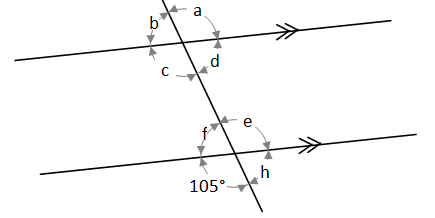Solution:

a = 105° because a = e (corresponding angles)

b = 75° because b = 180° – a (supplementary angles)

c = 105° because a = c (opposite angles)

d = 75° because d = b (opposite angles)

e = 105° because e = 105° (opposite angles).

f = 75° because f = 180° – e (supplementary angles)

h = 75° because h = 180° – 105° (supplementary angles)

Example 2: Find the following missing angles.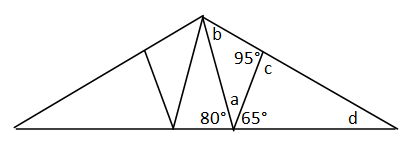Solution:

Find angle a:

a = 35° because 80° + 65° + a = 180° (supplementary angles)

a = 180° – 145°

or a = 35°

Find angle b:

b = 50° because 35° + 95° + b = 180° (sum of angles in a triangle)

b = 180° – 130°

or b = 60°

Find for c:

c = 85° because c + 95° = 180° (supplementary angles)

or c = 180° – 95° = 85°

Find for d:

d = 30° because 65° + 85° + c = 180° (sum of angles in a triangle)

d = 180° – 150° = 30°

Example 3: Find the unknown angles from the figure below.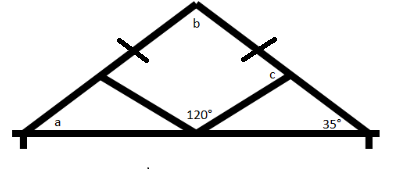Solution:

The triangle given is an isosceles triangle. The two base angles will be equal.

Hence a = 350

The two angles of the triangle are known. Adding the third angle makes 1800.

35° + 35° + b = 180°

b = 180° – 70°

b = 110°

The two angles of a quadrilateral are known. All four angles sum to 3600.

2c + 110° + 120° = 360°

2c = 360° – 230°

2c = 130°

c = 65°

Example 4: In the diagram given below, the lines l₁ and l₂ are parallel and line T is transversal. Find ∠GDE.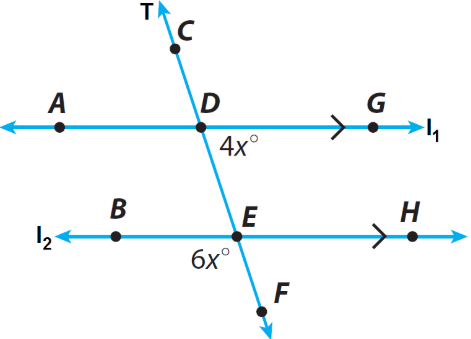Solution:

Step 1 :

In the above diagram, m∠GDE and m∠ADE are the angles on the straight line l₁.

So, we have

m∠GDE + m∠ADE = 180° —– (1)

Step 2 :

In the above diagram, m∠ADE and m∠BEF are corresponding angles and corresponding angles are always congruent.

So, we have

Step 3 :

In (1) replace m∠ADE by m∠BEF

(1) —–> m∠GDE + m∠BEF = 180°

Step 4 :

From the diagram given above, we have m∠GDE = 4x and m∠BEF = 6x. So, replace m∠GDE by 4x and m∠BEF by 6x.

4x + 6x = 180°

Combine like terms.

10x = 180°

Divide both sides by 10.

10x/10 = 180°/10

Simplify.

x = 18°

Step 5 :

Plug x = 18° in m∠GDE = 4x

m∠GDE = 4 · 18°

m∠GDE = 72°

Example 5: The measures of two angles of a triangle are $\text{6}0{}^\circ \text{ 53}’\text{ 51}”$ and $\text{51}{}^\circ \text{ 22}’\text{ 5}0”$ respectively. The measure of third angle in radian is____.

(e) None of these

Solution:

The sum of two angles $=[(\text{62}”\text{ 53}’\text{ 51}”)+\text{51}{}^\circ \text{22}’\text{ 5}0”]=\text{114}{}^\circ \text{ 16}’\text{ 41}”$

Third angle of triangle $=\text{18}0{}^\circ -(\text{14}0{}^\circ \text{ 16}’\text{ 41}”) =\text{65}{}^\circ \text{ 43}’\text{ 19}”\\ ={{\left( 65+\frac{43}{60}+\frac{19}{3600} \right)}^{0}}=\frac{234000+2580+19}{3600}={{\left( \frac{236599}{3600} \right)}^{0}}={{(65.722)}^{0}}$

We know that

${{1}^{o}}=\frac{\pi }{180} \text \ radian \text{(65}.\text{722}){}^\circ =\left( \frac{\pi }{180}\times 65.722 \right) \text \ radian = 1.15 \text \ radian\\$

Example 6: Find the ratio of radii of two circles, if arc of equal length subtend angles $\text{3}0{}^\circ$ and $\text{45}{}^\circ$ at their centre.

(a) 2:3

(b) 4:3

(c) 3:2

(d) 3 :4

(e) None of these

Solution:

Suppose radii of circles be ${{r}_{1}} \text \ and \ {{r}_{2}}$ then ${{\theta }_{1}}=\frac{l}{{{r}_{1}}} \text \ and \ {{\theta }_{2}}=\frac{l}{{{r}_{2}}}$ where

${{\theta }_{1}}={{30}^{o}},{{\theta }_{2}}={{45}^{o}}\\ \frac{{{\theta }_{1}}}{{{\theta }_{2}}}=\frac{\frac{l}{{{r}_{1}}}}{\frac{l}{{{r}_{2}}}}\\ \Rightarrow \frac{{{\theta }_{1}}}{{{\theta }_{2}}}=\frac{{{r}_{2}}}{{{r}_{1}}} \Rightarrow \frac{{{r}_{1}}}{{{r}_{2}}}=\frac{{{\theta }_{2}}}{{{\theta }_{1}}}\Rightarrow \frac{{{r}_{1}}}{{{r}_{2}}}=\frac{45}{30} \Rightarrow \frac{{{r}_{1}}}{{{r}_{2}}}=\frac{3}{2}\\ {{r}_{1}}:{{r}_{2}}=3:2\\$

Example 7: The perimeter of a sector of a circle of area 64 π sq. cm is 56 cm, then area of sector is

(A) 140 sq.cm

(B) 150 sq.cm

(C) 160 sq.cm

(D) 170 sq.cm

Solution:

A = 64 π

$\pi r^{2}$ = 64π

∴ r = 8 cm

Since, perimeter of sector = 2r + S

∴ S + r + r = 56

∴ S + 8 + 8 = 56

∴ S = 40

Since, S = r $\Theta$

∴ 40 = 8 × $\Theta$

∴ θ = 5c

∴ Area of sector = $\frac{1}{2}r^{2} \Theta = \frac{1}{2}[8^{2} *5]=160$ sq.cm.